Do you like the article?
Share it with others -

Use new possibilities of MetaTrader 5

# FANN2MQL Neural Network Tutorial

26 November 2009, 08:5422
12 035

### First of all:

Please install the fann2MQL Library. it is required to test this example. It can be dowloaded here.

### Introduction

Until now, there was only one example of how to use the Fann2MQL Library, which allow traders to use the Open-Source Neural Network Library "FANN" in their MQL codes.

But the example, written by the creator of the Fann2MQL Library, is not easy to understand. It's not made for beginners.

So I have written another example, way easier in its concept, and fully commented.

Iit is not related to trading directly, and is not using any financial data. It is a simple static example of application.

In this example, we are gonna teach a simple neural network to recognize a simple pattern:

The pattern we will teach if composed of 3 numbers: a, b and c.

• if a < b && b < c then expected output = 1
• if a < b && b > c then expected output = 0
• if a > b && b > c then expected output = 0
• if a > b && b < c then expected output = 1

You can think of those numbers as being vector coordinates for example (vector going up or down) or market direction. In which case, the pattern could be interpreted as:

UP UP = UP

UP DOWN = DOWN

DOWN DOWN = DOWN

DOWN UP = UP

First, we will create a neural network.

Then we are going to show the network some examples of patterns, so it can learn and deduce the rules.

Finally, we are going to show the network new patterns it has never seen, and ask him what are his conclusions. If he understood the rules, then he will be able to recognize those patterns.

### The commented code:

```// We include the Fann2MQl library
#include <Fann2MQL.mqh>

#property  indicator_separate_window
#property  indicator_buffers 0

// the total number of layers. here, there is one input layer,
// 2 hidden layers, and one output layer = 4 layers.
int nn_layer = 4;
int nn_input = 3; // Number of input neurones. Our pattern is made of 3 numbers,
// so that means 3 input neurones.
int nn_hidden1 = 8; // Number of neurones on the first hidden layer
int nn_hidden2 = 5; // number on the second hidden layer
int nn_output = 1; // number of outputs

// trainingData[][] will contain the examples
// we're gonna use to teach the rules to the neurones.
double      trainingData[];  // IMPORTANT! size = nn_input + nn_output

int maxTraining = 500;  // maximum number of time we will train
// the neurones with some examples
double targetMSE = 0.002; // the Mean-Square Error of the neurones we should
// get at most (you will understand this lower in the code)

int ann; // This var will be the identifier of the neuronal network.

// When the indicator is removed, we delete all of the neurnal networks
// from the memory of the computer.
int deinit() {
f2M_destroy_all_anns();
return(0);
}

int init() {
int i;
double MSE;

Print("=================================== START EXECUTION ================================");

IndicatorBuffers(0);
IndicatorDigits(6);

// We resize the trainingData array, so we can use it.
// We're gonna change its size one size at a time.
ArrayResize(trainingData,1);

Print("##### INIT #####");

// We create a new neuronal networks
ann = f2M_create_standard(nn_layer, nn_input, nn_hidden1, nn_hidden2, nn_output);

// we check if it was created successfully. 0 = OK, -1 = error
debug("f2M_create_standard()",ann);

// We set the activation function. Don't worry about that. Just do it.
f2M_set_act_function_hidden (ann, FANN_SIGMOID_SYMMETRIC_STEPWISE);
f2M_set_act_function_output (ann, FANN_SIGMOID_SYMMETRIC_STEPWISE);

// Some studies show that statistically, the best results are reached using this range;
// but you can try to change and see is it gets better or worst
f2M_randomize_weights (ann, -0.77, 0.77);

// I just print to the console the number of input and output neurones.
// Just to check. Just for debug purpose.
debug("f2M_get_num_input(ann)",f2M_get_num_input(ann));
debug("f2M_get_num_output(ann)",f2M_get_num_output(ann));

Print("##### REGISTER DATA #####");

// Now we prepare some data examples (with expected output)
// and we add them to the training set.
// Once we have add all the examples we want, we're gonna send
// this training data set to the neurones, so they can learn.
// prepareData() has a few arguments:
// - Action to do (train or compute)
// - the data (here, 3 data per set)
// - the last argument is the expected output.
// Here, this function takes the example data and the expected output,
// and add them to the learning set.
// Check the comment associated with this function to get more details.
//
// here is the pattern we're going to teach:
// There is 3 numbers. Let's call them a, b and c.
// You can think of those numbers as being vector coordinates
// for example (vector going up or down)
// if a < b && b < c then output = 1
// if a < b && b > c then output = 0
// if a > b && b > c then output = 0
// if a > b && b < c then output = 1

// UP UP = UP / if a < b && b < c then output = 1
prepareData("train",1,2,3,1);
prepareData("train",8,12,20,1);
prepareData("train",4,6,8,1);
prepareData("train",0,5,11,1);

// UP DOWN = DOWN / if a < b && b > c then output = 0
prepareData("train",1,2,1,0);
prepareData("train",8,10,7,0);
prepareData("train",7,10,7,0);
prepareData("train",2,3,1,0);

// DOWN DOWN = DOWN / if a > b && b > c then output = 0
prepareData("train",8,7,6,0);
prepareData("train",20,10,1,0);
prepareData("train",3,2,1,0);
prepareData("train",9,4,3,0);
prepareData("train",7,6,5,0);

// DOWN UP = UP / if a > b && b < c then output = 1
prepareData("train",5,4,5,1);
prepareData("train",2,1,6,1);
prepareData("train",20,12,18,1);
prepareData("train",8,2,10,1);

// Now we print the full training set to the console, to check how it looks like.
// this is just for debug purpose.
printDataArray();

Print("##### TRAINING #####");

// We need to train the neurones many time in order
// for them to be good at what we ask them to do.
// Here I will train them with the same data (our examples) over and over again,
// until they fully understand the rules we are trying to teach them, or until
// the training has been repeated 'maxTraining' number of time
// (in this case maxTraining = 500)
// The better they understand the rule, the lower their mean-Square Error will be.
// the teach() function returns this mean-Square Error (or MSE)
// 0.1 or lower is a sufficient number for simple rules
// 0.02 or lower is better for complex rules like the one
// we are trying to teach them (it's a patttern recognition. not so easy.)
for (i=0;i<maxTraining;i++) {
MSE = teach(); // everytime the loop run, the teach() function is activated.
// Check the comments associated to this function to understand more.
if (MSE < targetMSE) { // if the MSE is lower than what we defined (here targetMSE = 0.02)
debug("training finished. Trainings ",i+1); // then we print in the console
// how many training
// it took them to understand
i = maxTraining; // and we go out of this loop
}
}

// we print to the console the MSE value once the training is completed
debug("MSE",f2M_get_MSE(ann));

Print("##### RUNNING #####");
// And now we can ask the neurone to analyse new data that they never saw.
// Will they recognize the patterns correctly?
// You can see that I used the same prepareData() function here,
// with the first argument set to "compute".
// The last argument which was dedicated to the expected output
// when we used this function for registering examples earlier,
// is now useless, so we leave it to zero.
// if you prefer, you can call directly the compute() function.
// In this case, the structure is compute(inputVector[]);
// So instead of prepareData("compute",1,3,1,0); you would do something like:
//    double inputVector[]; // declare a new array
//    ArrayResize(inputVector,f2M_get_num_input(ann));
// resize the array to the number of neuronal input
//    inputVector = 1; // add in the array the data
//    inputVector = 3;
//    inputVector = 1;
//    result = compute(inputVector); // call the compute() function, with the input array.
// the prepareData() function call the compute() function,
// which print the result to the console,
// so we can check if the neurones were right or not.
debug("1,3,1 = UP DOWN = DOWN. Should output 0.","");
prepareData("compute",1,3,1,0);

debug("1,2,3 = UP UP = UP. Should output 1.","");
prepareData("compute",1,2,3,0);

debug("3,2,1 = DOWN DOWN = DOWN. Should output 0.","");
prepareData("compute",3,2,1,0);

debug("45,2,89 = DOWN UP = UP. Should output 1.","");
prepareData("compute",45,2,89,0);

debug("1,3,23 = UP UP = UP. Should output 1.","");
prepareData("compute",1,3,23,0);

debug("7,5,6 = DOWN UP = UP. Should output 1.","");
prepareData("compute",7,5,6,0);

debug("2,8,9 = UP UP = UP. Should output 1.","");
prepareData("compute",2,8,9,0);

Print("=================================== END EXECUTION ================================");
return(0);
}

int start() {
return(0);
}

/*************************
** printDataArray()
** Print the datas used for training the neurones
** This is useless. Just created for debug purpose.
*************************/
void printDataArray() {
int i,j;
int bufferSize = ArraySize(trainingData)/(f2M_get_num_input(ann)+f2M_get_num_output(ann))-1;
string lineBuffer = "";
for (i=0;i<bufferSize;i++) {
for (j=0;j<(f2M_get_num_input(ann)+f2M_get_num_output(ann));j++) {
lineBuffer = StringConcatenate(lineBuffer, trainingData[i][j], ",");
}
debug("DataArray["+i+"]", lineBuffer);
lineBuffer = "";
}
}

/*************************
** prepareData()
** Prepare the data for either training or computing.
** It takes the data, put them in an array,
** and send them to the training or running function
** Update according to the number of input/output your code needs.
*************************/
void prepareData(string action, double a, double b, double c, double output) {
double inputVector[];
double outputVector[];
// we resize the arrays to the right size
ArrayResize(inputVector,f2M_get_num_input(ann));
ArrayResize(outputVector,f2M_get_num_output(ann));

inputVector = a;
inputVector = b;
inputVector = c;
outputVector = output;
if (action == "train") {
}
if (action == "compute") {
compute(inputVector);
}
// if you have more input than 3, just change the structure of this function.
}

/*************************
** Add a single set of training data
**(data example + expected output) to the global training set
*************************/
void addTrainingData(double inputArray[], double outputArray[]) {
int j;
int bufferSize = ArraySize(trainingData)/(f2M_get_num_input(ann)+f2M_get_num_output(ann))-1;

//register the input data to the main array
for (j=0;j<f2M_get_num_input(ann);j++) {
trainingData[bufferSize][j] = inputArray[j];
}
for (j=0;j<f2M_get_num_output(ann);j++) {
trainingData[bufferSize][f2M_get_num_input(ann)+j] = outputArray[j];
}

ArrayResize(trainingData,bufferSize+2);
}

/*************************
** teach()
** Get all the trainign data and use them to train the neurones one time.
** In order to properly train the neurones, you need to run ,
** this function many time until the Mean-Square Error get low enough.
*************************/
double teach() {
int i,j;
double MSE;
double inputVector[];
double outputVector[];
ArrayResize(inputVector,f2M_get_num_input(ann));
ArrayResize(outputVector,f2M_get_num_output(ann));
int call;
int bufferSize = ArraySize(trainingData)/(f2M_get_num_input(ann)+f2M_get_num_output(ann))-1;
for (i=0;i<bufferSize;i++) {
for (j=0;j<f2M_get_num_input(ann);j++) {
inputVector[j] = trainingData[i][j];
}
outputVector = trainingData[i];
//f2M_train() is showing the neurones only one example at a time.
call = f2M_train(ann, inputVector, outputVector);
}
// Once we have show them an example,
// we check if how good they are by checking their MSE.
// If it's low, they learn good!
MSE = f2M_get_MSE(ann);
return(MSE);
}

/*************************
** compute()
** Compute a set of data and returns the computed result
*************************/
double compute(double inputVector[]) {
int j;
int out;
double output;
ArrayResize(inputVector,f2M_get_num_input(ann));

// We sent new data to the neurones.
out = f2M_run(ann, inputVector);
// and check what they say about it using f2M_get_output().
output = f2M_get_output(ann, 0);
debug("Computing()",MathRound(output));
return(output);
}

/*************************
** debug()
** Print data to the console
*************************/
void debug(string a, string b) {
Print(a+" ==> "+b);
}```

### The output

Output of the Neural network in the console.

### Conclusion

Also you can read the article "Using Neural Networks In MetaTrader" written by Mariusz Woloszyn, author of the Fann2MQL Library.

It took me 4 days to understand how to use Fann in MetaTrader, by analyzing the little documentation that is available here and on google.

I hope this example will be useful for you, and that it will avoid you to loose too much time experimenting. More articles will follow in the next few weeks.

Attached files |
ASNN_1_learner.mq4 (11.74 KB)
Last comments | Go to discussion (22)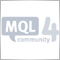| 7 Oct 2010 at 10:07

hey, i've got a problem, when i change the number of output, it doesn't work.
Even if i change the structure of the preparData function, the output number still 1
Can someone help me please ?| 8 Dec 2011 at 07:48

Hallo,

is it possible to get 2 and 3 as output for 0 and 1?

When I train it, everything seems to be OK.

But when I compute it, the output every time returns 1.

EURUSD,M15: Computing() ==> 1.00000000

EURUSD,M15: Computing() ==> 1.00000000 2011.12.08 08:34:41 ASNN 1 learner(1) EURUSD,M15: 45,2,89 = DOWN UP = UP. Should output 2. ==>

EURUSD,M15: Computing() ==> 1.00000000

EURUSD,M15: Computing() ==> 1.00000000 2011.12.08 08:34:41 ASNN 1 learner(1) EURUSD,M15: 3,2,1 = DOWN DOWN = DOWN. Should output 3. ==>| 20 Dec 2016 at 04:55

Thank you very much for creating and delivering FANN2MQL package, could you please add more detailed descriptions for it's subroutines, maybe in a reference manual?

for example, I have some questions about the usage of subroutine

int f2M_create_standard(int num_layers, int l1num, int l2num, int l3num, int l4num);

to create an ANN:

1. How many layers can be created? maximum 3 hidden layers, or can be arbitrary?

2. In the opposite direction, I tried to create a simple ANN with only 1 hidden layer with f2m_create_standard(3, 10, 100, 1); but it refuse to eat saying an error "wrong parameter count". What was my mistake? or it does not support single hidden layer at all?| 20 Jan 2018 at 09:59

I don't know if this thread is still active.
But i got a question.

As far as running the program, that worked.
But when closer investigation of the program,

i found out that the dependencies didn't all load.
of the 6 dll's that are required, only 1 of them (Fann2MQL.dll) loads.

just to clarify, it's about tbb.dll, kernel32.dll, fanndouble.dll, user32.dll and msvcr100.dll

I know for a fact that all of the dll's are on my laptop.

I even went out of my way to install the tbb.dll from intel website.

I was wondering who else had that issue and who managed to fix it.
If fixed, how.

kind regards| 21 Jan 2018 at 13:30

I managed to to get it working a year or two ago. Even with tbb which surprised me a that time. But don't recall now if I had to recompile the dll or what. I used then that as a basis to try different parallel execution ways. That code includes also ways to run several threads without tbb but at least I didn't get those working in my (different case). It's somewhat a nightmare to get dll dependencies so that MT4 accepts it.. and with tbb I had hard time as well. If you haven't tried try some dll dependency tools on the internet. My observation is that if your dll depends on some other dll MT4 wont load it and your MT4  execution stops to your first call to your dll. If you managed to run the example you are either not calling any routine from the dll at all or the dll dependencies are ok.

It's also quite hard to know which version of those tbb dlls to install ... debug, x64, x86, win, release nbr.

Hope this helps.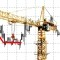Trader's Kit: Decorating Indicators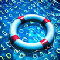Working with Doubles in MQL4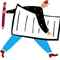Meta COT Project - New Horizons for CFTC Report Analysis in MetaTrader 4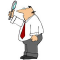Checking the Myth: The Whole Day Trading Depends on How the Asian Session Is Traded